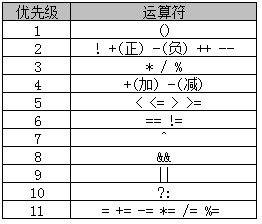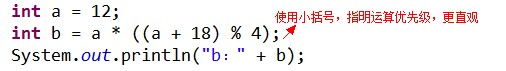# Java中常用的运算符

## 运算符定义

Java 语言中常用的运算符可分为如下几种：

1. 算术运算符
2. 赋值运算符
3. 比较运算符
4. 逻辑运算符
5. 条件运算符

 12345678 public class Addition{     public static void main(String[] args) {         int a=12;         int b=24;         int sum = a+b;         System.out.println("两数之和为："+sum);     } }

## Java算术运算符

+ 加法 – 相加运算符两侧的值 A + B 等于 30

* 乘法 – 相乘操作符两侧的值 A * B等于200
/ 除法 – 左操作数除以右操作数 B / A等于2

++ 自增: 操作数的值增加1 B++ 或 ++B 等于 21（区别详见下文）

 1234567891011121314151617181920 public class Test {   public static void main(String args[]) {      int a = 10;      int b = 20;      int c = 25;      int d = 25;      System.out.println("a + b = " + (a + b) );      System.out.println("a - b = " + (a - b) );      System.out.println("a * b = " + (a * b) );      System.out.println("b / a = " + (b / a) );      System.out.println("b % a = " + (b % a) );      System.out.println("c % a = " + (c % a) );      System.out.println("a++   = " +  (a++) );      System.out.println("a--   = " +  (a--) );      // 查看  d++ 与 ++d 的不同      System.out.println("d++   = " +  (d++) );      System.out.println("++d   = " +  (++d) );   } }

 12345678910 a + b = 30 a - b = -10 a * b = 200 b / a = 2 b % a = 0 c % a = 5 a++   = 10 a--   = 11 d++   = 25 ++d   = 27

### 自增自减运算符

1、自增（++）自减（–）运算符是一种特殊的算术运算符，在算术运算符中需要两个操作数来进行运算，而自增自减运算符是一个操作数。

 12345678910 public class selfAddMinus{     public static void main(String[] args){         int a = 3;//定义一个变量；         int b = ++a;//自增运算         int c = 3;         int d = --c;//自减运算         System.out.println("进行自增运算后的值等于"+b);         System.out.println("进行自减运算后的值等于"+d);     } }

 12 进行自增运算后的值等于4 进行自减运算后的值等于2

1. int b = ++a; 拆分运算过程为: a=a+1=4; b=a=4, 最后结果为b=4,a=4
2. int d = –c; 拆分运算过程为: c=c-1=2; d=c=2, 最后结果为d=2,c=2

2、前缀自增自减法(++a,–a): 先进行自增或者自减运算，再进行表达式运算。

3、后缀自增自减法(a++,a–): 先进行表达式运算，再进行自增或者自减运算 实例：

 12345678910 public class selfAddMinus{     public static void main(String[] args){         int a = 5;//定义一个变量；         int b = 5;         int x = 2*++a;         int y = 2*b++;         System.out.println("自增运算符前缀运算后a="+a+",x="+x);         System.out.println("自增运算符后缀运算后b="+b+",y="+y);     } }

 12 自增运算符前缀运算后a=6，x=12 自增运算符后缀运算后b=6，y=10

PS：% 用来求余数，也称为”取模运算符“

## Java赋值运算符

= 简单的赋值运算符，将右操作数的值赋给左侧操作数 C = A + B将把A + B得到的值赋给C
+ = 加和赋值操作符，它把左操作数和右操作数相加赋值给左操作数 C + = A等价于C = C + A
– = 减和赋值操作符，它把左操作数和右操作数相减赋值给左操作数 C – = A等价于C = C –
A
* = 乘和赋值操作符，它把左操作数和右操作数相乘赋值给左操作数 C * = A等价于C = C * A
/ = 除和赋值操作符，它把左操作数和右操作数相除赋值给左操作数 C / = A等价于C = C / A
％= 取模和赋值操作符，它把左操作数和右操作数取模后赋值给左操作数 C％= A等价于C = C％A
<< = 左移位赋值运算符 C << = 2等价于C = C << 2
>> = 右移位赋值运算符 C >> = 2等价于C = C >> 2
＆= 按位与赋值运算符 C＆= 2等价于C = C＆2
^ = 按位异或赋值操作符 C ^ = 2等价于C = C ^ 2
| = 按位或赋值操作符 C | = 2等价于C = C | 2

*上表中粉色表格内容代表Java 支持的常用赋值运算符

 1234567891011121314151617181920212223242526272829303132333435 public class Test {   public static void main(String[] args) {      int a = 10;      int b = 20;      int c = 0;      c = a + b;      System.out.println("c = a + b = " + c );      c += a ;      System.out.println("c += a  = " + c );      c -= a ;      System.out.println("c -= a = " + c );      c *= a ;      System.out.println("c *= a = " + c );      a = 10;      c = 15;      c /= a ;      System.out.println("c /= a = " + c );      a = 10;      c = 15;      c %= a ;      System.out.println("c %= a  = " + c );      c <<= 2 ;      System.out.println("c <<= 2 = " + c );      c >>= 2 ;      System.out.println("c >>= 2 = " + c );      c >>= 2 ;      System.out.println("c >>= a = " + c );      c &= a ;      System.out.println("c &= 2  = " + c );      c ^= a ;      System.out.println("c ^= a   = " + c );      c |= a ;      System.out.println("c |= a   = " + c );   } }

 123456789101112 c = a + b = 30 c += a  = 40 c -= a = 30 c *= a = 300 c /= a = 1 c %= a  = 5 c <<= 2 = 20 c >>= 2 = 5 c >>= 2 = 1 c &= a  = 0 c ^= a   = 10 c |= a   = 10

## Java比较运算符

Java 中常用的比较运算符如下表所示：

== 检查如果两个操作数的值是否相等，如果相等则条件为真。 （A == B）false
!= 检查如果两个操作数的值是否相等，如果值不相等则条件为真。 (A != B) true
> 检查左操作数的值是否大于右操作数的值，如果是那么条件为真。 （A> B）true
< 检查左操作数的值是否小于右操作数的值，如果是那么条件为真。 （A <B）true
>= 检查左操作数的值是否大于或等于右操作数的值，如果是那么条件为真。 （A> = B）false
<= 检查左操作数的值是否小于或等于右操作数的值，如果是那么条件为真。 （A <= B）true

### 实例

 12345678910111213 public class Test {     public static void main(String[] args) {      int a = 10;      int b = 20;      System.out.println("a == b = " + (a == b) );      System.out.println("a != b = " + (a != b) );      System.out.println("a > b = " + (a > b) );      System.out.println("a < b = " + (a < b) );      System.out.println("b >= a = " + (b >= a) );      System.out.println("b <= a = " + (b <= a) );   } }

 123456 a == b = false a != b = true a > b = false a < b = true b >= a = true b <= a = false

1、 > 、 < 、 >= 、 <= 只支持左右两边操作数是数值类型

2、 == 、 != 两边的操作数既可以是数值类型，也可以是引用类型

## Java逻辑运算符

&& 称为逻辑与运算符。当且仅当两个操作数都为真，条件才为真。 （A && B）为 false
| | 称为逻辑或操作符。如果任何两个操作数任何一个为真，条件为真。 （A | | B）为 true

### 实例

 123456789 public class Test {   public static void main(String[] args) {      boolean a = true;      boolean b = false;      System.out.println("a && b = " + (a&&b));      System.out.println("a || b = " + (a||b) );      System.out.println("!(a && b) = " + !(a && b));   } }

 1234567891011121314151617181920 a && b = false a || b = true !(a && b) = true 当使用逻辑运算符时，我们会遇到一种很有趣的“短路”现象。 譬如：( one > two ) && ( one < three ) 中，如果能确定左边 one > two 运行结果为 false , 则系统就认为已经没有必要执行右侧的 one < three 啦。 同理，在( one > two ) || ( one < three ) 中，如果能确定左边表达式的运行结果为 true , 则系统也同样会认为已经没有必要再进行右侧的 one < three 的执行啦！ 当使用与逻辑运算符时，在两个操作数都为true时，结果才为true，但是当得到第一个操作为false时，其结果就必定是false，这时候就不会再判断第二个操作了。 实例： [cc lang="html"]public class LuoJi{     public static void main(String[] args){         int a = 5;//定义一个变量；         boolean b = (a<4)&&(a++<10);         System.out.println("使用短路逻辑运算符的结果为"+b);         System.out.println("a的结果为"+a);     } }

 12 使用短路逻辑运算符的结果为false a的结果为5

## Java条件运算符

 1 variable x = (expression) ? value if true : value if false

 12345678910111213 public class Test {    public static void main(String[] args){       int a , b;       a = 10;       // 如果 a 等于 1 成立，则设置 b 为 20，否则为 30       b = (a == 1) ? 20 : 30;       System.out.println( "Value of b is : " +  b );         // 如果 a 等于 10 成立，则设置 b 为 20，否则为 30       b = (a == 10) ? 20 : 30;       System.out.println( "Value of b is : " + b );    } }

 12 Value of b is : 30 Value of b is : 20

## Java中运算符的优先级PS：大家没必要去死记运算符的优先级顺序，实际开发中，一般会使用小括号辅助进行优先级管理。例如：1、 执行 a + 18 ，结果为 30

2、 执行( a + 18 ) % 4 取模，结果为 2

3、 执行 a * ( ( a + 18 ) % 4 )，结果为 24

### 觉得文章有用就打赏一下文章作者

#### 支付宝扫一扫打赏#### 微信扫一扫打赏### 在线客服

•扫描二维码，微信联系